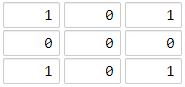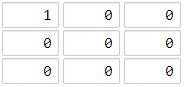1162. As Far from Land as Possible
Medium
3.8K
98

Given an `n x n` `grid` containing only values `0` and `1`, where `0` represents water and `1` represents land, find a water cell such that its distance to the nearest land cell is maximized, and return the distance. If no land or water exists in the grid, return `-1`.

The distance used in this problem is the Manhattan distance: the distance between two cells `(x0, y0)` and `(x1, y1)` is `|x0 - x1| + |y0 - y1|`.

Example 1:```Input: grid = [[1,0,1],[0,0,0],[1,0,1]]
Output: 2
Explanation: The cell (1, 1) is as far as possible from all the land with distance 2.
```

Example 2:```Input: grid = [[1,0,0],[0,0,0],[0,0,0]]
Output: 4
Explanation: The cell (2, 2) is as far as possible from all the land with distance 4.
```

Constraints:

• `n == grid.length`
• `n == grid[i].length`
• `1 <= n <= 100`
• `grid[i][j]` is `0` or `1`
Accepted
136.1K
Submissions
262.2K
Acceptance Rate
51.9%

Seen this question in a real interview before?
1/4
Yes
No

Discussion (0)

Related Topics